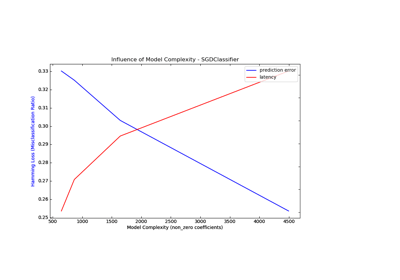/scikit-learn

# sklearn.metrics.hamming_loss

`sklearn.metrics.hamming_loss(y_true, y_pred, labels=None, sample_weight=None)` [source]

Compute the average Hamming loss.

The Hamming loss is the fraction of labels that are incorrectly predicted.

Read more in the User Guide.

Parameters: `y_true : 1d array-like, or label indicator array / sparse matrix` Ground truth (correct) labels. `y_pred : 1d array-like, or label indicator array / sparse matrix` Predicted labels, as returned by a classifier. `labels : array, shape = [n_labels], optional (default=None)` Integer array of labels. If not provided, labels will be inferred from y_true and y_pred. New in version 0.18. `sample_weight : array-like of shape = [n_samples], optional` Sample weights. New in version 0.18. `loss : float or int,` Return the average Hamming loss between element of `y_true` and `y_pred`.

#### Notes

In multiclass classification, the Hamming loss correspond to the Hamming distance between `y_true` and `y_pred` which is equivalent to the subset `zero_one_loss` function.

In multilabel classification, the Hamming loss is different from the subset zero-one loss. The zero-one loss considers the entire set of labels for a given sample incorrect if it does entirely match the true set of labels. Hamming loss is more forgiving in that it penalizes the individual labels.

The Hamming loss is upperbounded by the subset zero-one loss. When normalized over samples, the Hamming loss is always between 0 and 1.

#### References

  Grigorios Tsoumakas, Ioannis Katakis. Multi-Label Classification: An Overview. International Journal of Data Warehousing & Mining, 3(3), 1-13, July-September 2007.

#### Examples

```>>> from sklearn.metrics import hamming_loss
>>> y_pred = [1, 2, 3, 4]
>>> y_true = [2, 2, 3, 4]
>>> hamming_loss(y_true, y_pred)
0.25
```

In the multilabel case with binary label indicators:

```>>> hamming_loss(np.array([[0, 1], [1, 1]]), np.zeros((2, 2)))
0.75
```

## Examples using `sklearn.metrics.hamming_loss`Model Complexity Influence

© 2007–2018 The scikit-learn developers
Licensed under the 3-clause BSD License.
http://scikit-learn.org/stable/modules/generated/sklearn.metrics.hamming_loss.html